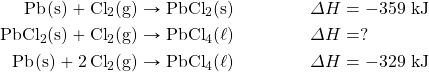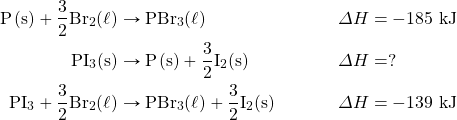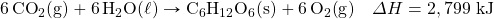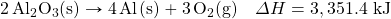Chapter 7. Energy and Chemistry

# End-of-Chapter Material

1. What is the work when 124 mL of gas contract to 72.0 mL under an external pressure of 822 torr?
2. What is the work when 2,345 mL of gas contract to 887 mL under an external pressure of 348 torr?
3. A 3.77 L volume of gas is exposed to an external pressure of 1.67 atm. As the gas contracts, 156 J of work are added to the gas. What is the final volume of the gas?
4. A 457 mL volume of gas contracts when 773 torr of external pressure act on it. If 27.4 J of work are added to the gas, what is its final volume?
5. What is the heat when 1,744 g of Hg increase in temperature by 334°C? Express your final answer in kJ.
6. What is the heat when 13.66 kg of Fe cool by 622°C? Express your final answer in kJ.
7. What is final temperature when a 45.6 g sample of Al at 87.3°C gains 188 J of heat?
8. What is final temperature when 967 g of Au at 557°C lose 559 J of heat?
9. Plants take CO2 and H2O and make glucose (C6H12O6) and O2. Write a balanced thermochemical equation for this process. Use data in Table 7.1 “Enthalpies of Formation for Various Substances.”
10. Exercise 9 described the formation of glucose in plants, which take in CO2 and H2O and give off O2. Is this process exothermic or endothermic? If exothermic, where does the energy go? If endothermic, where does the energy come from?
11. The basic reaction in the refining of aluminum is to take Al2O3(s) and turn it into Al(s) and O2(g). Write the balanced thermochemical equation for this process. Use data in Table 7.1.
12. Is the enthalpy change of the reaction H2O(ℓ) → H2O(g) zero or nonzero? Use data in Table 7.1 to determine the answer.
13. What mass of H2O can be heated from 22°C to 80°C in the combustion of 1 mol of CH4? You will need the balanced thermochemical equation for the combustion of CH4. Use data in Table 7.1.
14. What mass of H2O can be heated from 22°C to 80°C in the combustion of 1 mol of C2H6? You will need the balanced thermochemical equation for the combustion of C2H6. Use data in Table 7.1. Compare your answer to Exercise 13.
15. What is the enthalpy change for the unknown reaction?16. What is the enthalpy change for the unknown reaction?17. What is the ΔH for the reaction C(s, gra) → C(s, dia)? The label gra means graphite, and the label dia means diamond. What does your answer mean?
18. Without consulting any tables, determine the ΔH for the reaction H2O(ℓ, 25°C) → H2O(ℓ, 25°C). Explain your answer.

1. 5.70 J
1. 4.69 L
1. 80.97 kJ
1. 91.9°C
1.1.1. 3,668 g
1. ΔH = 30 kJ
1. ΔH = 1.897 kJ; the reaction is endothermic.